# Network chart with R and igraph: chart parameters

The `igraph` package is the best way to build network diagrams with R. This post describes the different parameters offered by the library, allowing to customize node, link and label features..

# Default network chart with `igraph`

This post assumes you already know how to build a basic network chart with the `igraph` library. If you don’t have a look to the network section that provides an introduction.

Basically, `igraph` allows to transform several types of input into a `graph` object that can be plotted using the `plot()` function as follow: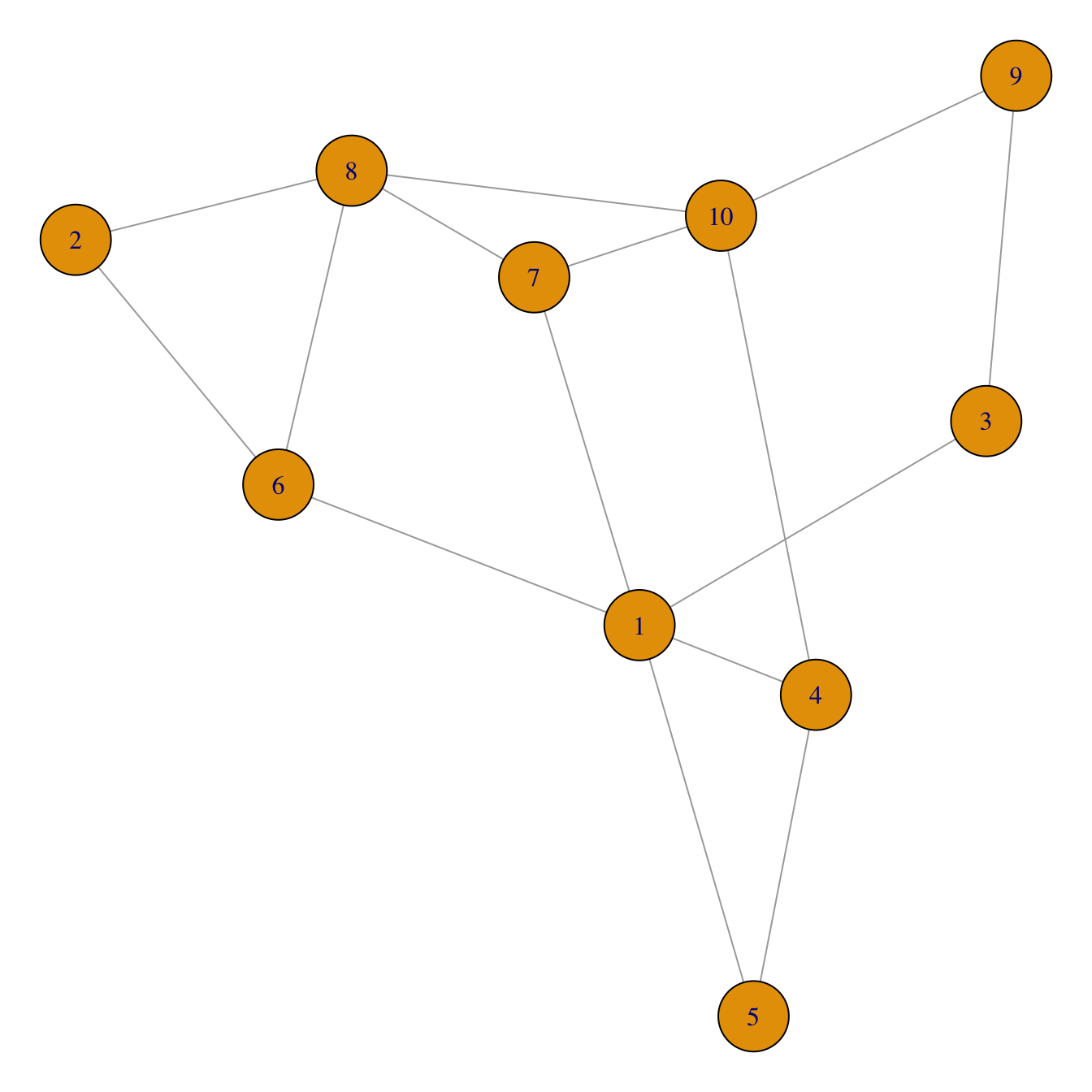``````# Library
library(igraph)

# Create data
set.seed(1)
data <- matrix(sample(0:1, 100, replace=TRUE, prob=c(0.8,0.2)), nc=10)
network <- graph_from_adjacency_matrix(data , mode='undirected', diag=F )

# Default network
par(mar=c(0,0,0,0))
plot(network)``````

# Customize node features

Node can be modified buy several arguments that all start with `vertex.`. A vertex is a node in `igraph` language. Main options are explained in the code below: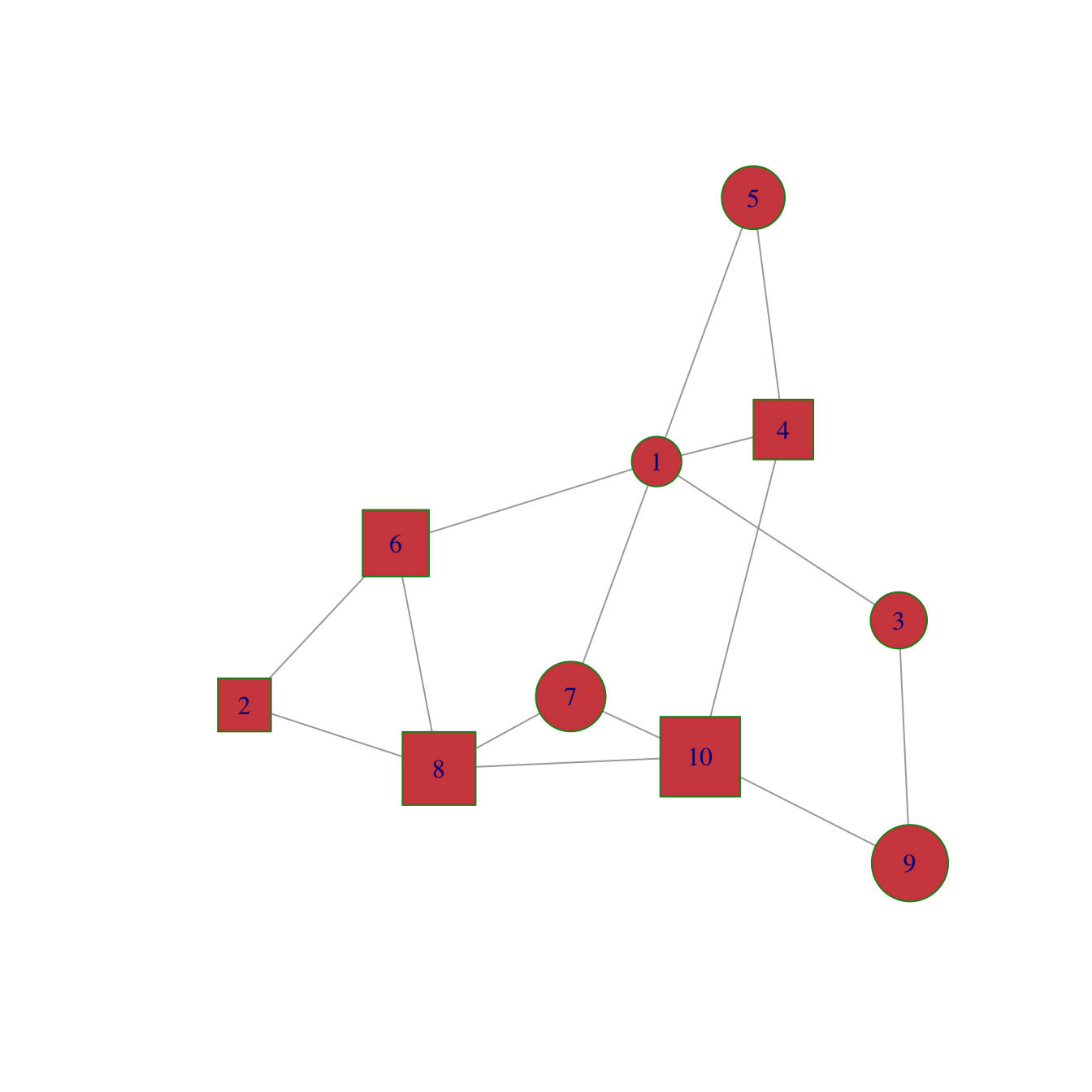``````plot(network,
vertex.color = rgb(0.8,0.2,0.2,0.9),           # Node color
vertex.frame.color = "Forestgreen",            # Node border color
vertex.shape=c("circle","square"),             # One of “none”, “circle”, “square”, “csquare”, “rectangle” “crectangle”, “vrectangle”, “pie”, “raster”, or “sphere”
vertex.size=c(15:24),                          # Size of the node (default is 15)
vertex.size2=NA,                               # The second size of the node (e.g. for a rectangle)
)``````

# Customize label features

Following the same principle, options starting with `vertex.label.` control the labgel features. Note that when several values are provided to an option, values are used subsequently and recycled.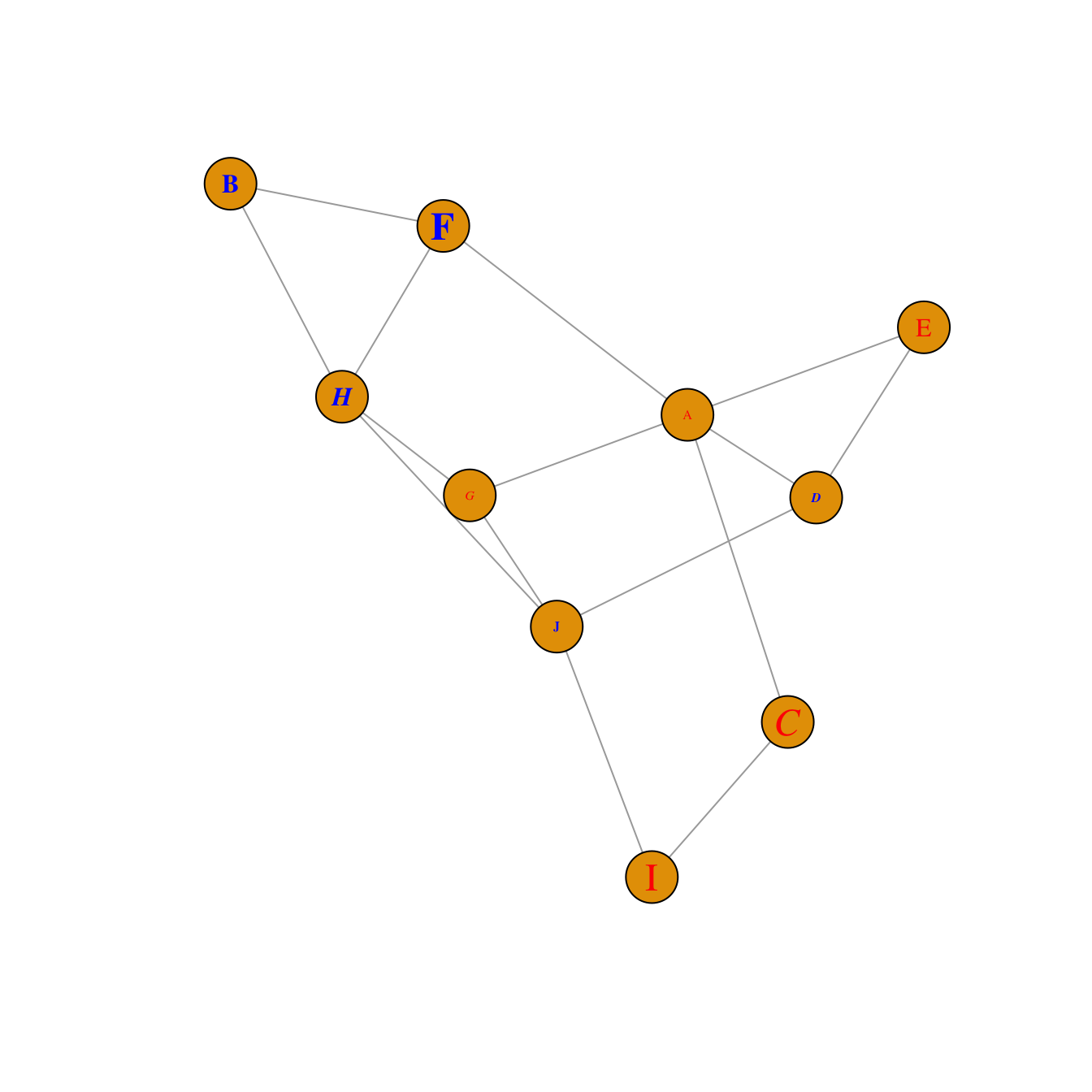``````plot(network,
vertex.label=LETTERS[1:10],                    # Character vector used to label the nodes
vertex.label.color=c("red","blue"),
vertex.label.family="Times",                   # Font family of the label (e.g.“Times”, “Helvetica”)
vertex.label.font=c(1,2,3,4),                  # Font: 1 plain, 2 bold, 3, italic, 4 bold italic, 5 symbol
vertex.label.cex=c(0.5,1,1.5),                 # Font size (multiplication factor, device-dependent)
vertex.label.dist=0,                           # Distance between the label and the vertex
vertex.label.degree=0 ,                        # The position of the label in relation to the vertex (use pi)
)``````

Last but not least, control edges with arguments starting with `edge.`.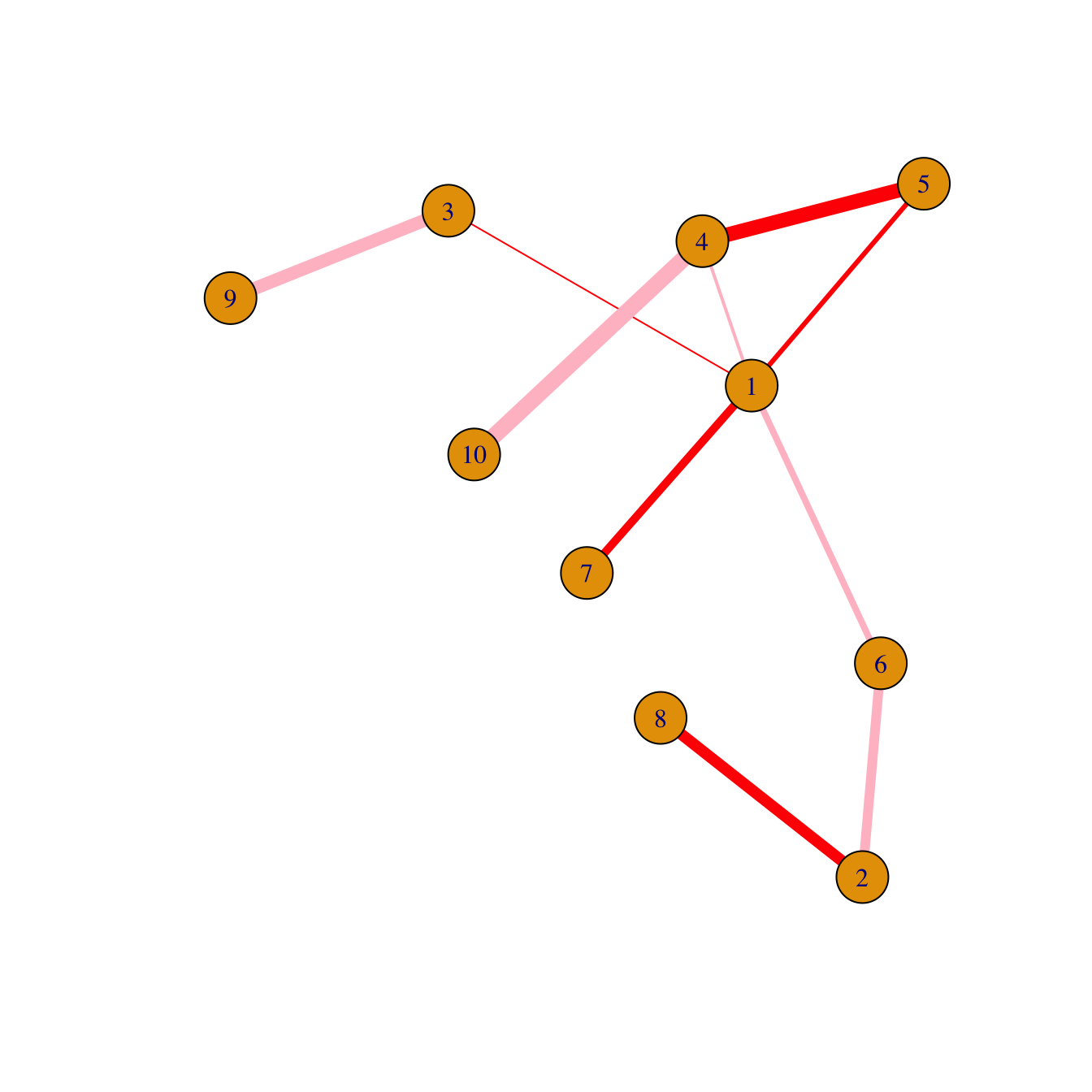``````plot(network,
edge.color=rep(c("red","pink"),5),           # Edge color
edge.width=seq(1,10),                        # Edge width, defaults to 1
edge.arrow.size=1,                           # Arrow size, defaults to 1
edge.arrow.width=1,                          # Arrow width, defaults to 1
edge.lty=c("solid")                           # Line type, could be 0 or “blank”, 1 or “solid”, 2 or “dashed”, 3 or “dotted”, 4 or “dotdash”, 5 or “longdash”, 6 or “twodash”
#edge.curved=c(rep(0,5), rep(1,5))            # Edge curvature, range 0-1 (FALSE sets it to 0, TRUE to 0.5)
)``````

# All customization

Of course, you can use all the options described above all together on the same chart, for a high level of customization.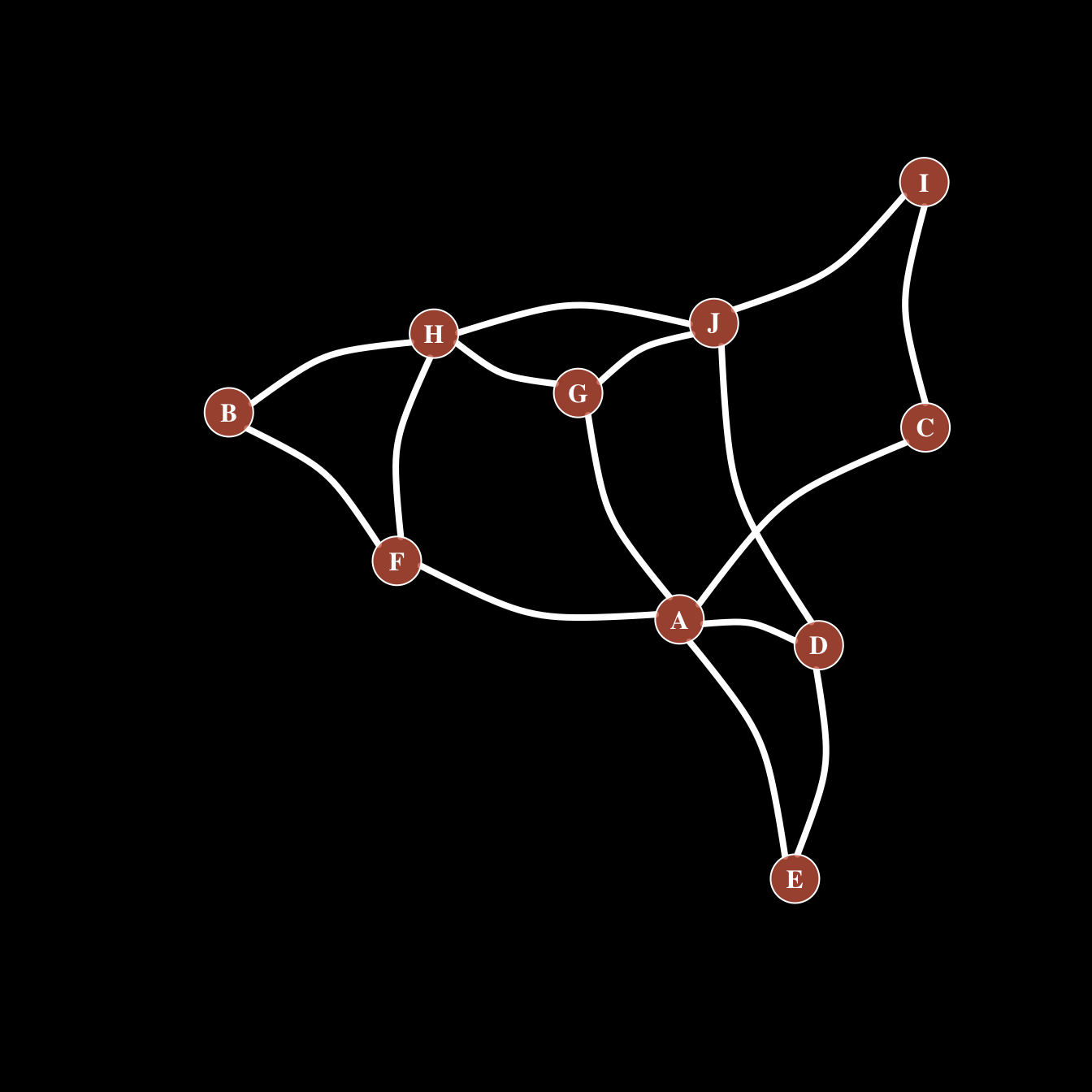``````par(bg="black")

plot(network,

# === vertex
vertex.color = rgb(0.8,0.4,0.3,0.8),          # Node color
vertex.frame.color = "white",                 # Node border color
vertex.shape="circle",                        # One of “none”, “circle”, “square”, “csquare”, “rectangle” “crectangle”, “vrectangle”, “pie”, “raster”, or “sphere”
vertex.size=14,                               # Size of the node (default is 15)
vertex.size2=NA,                              # The second size of the node (e.g. for a rectangle)

# === vertex label
vertex.label=LETTERS[1:10],                   # Character vector used to label the nodes
vertex.label.color="white",
vertex.label.family="Times",                  # Font family of the label (e.g.“Times”, “Helvetica”)
vertex.label.font=2,                          # Font: 1 plain, 2 bold, 3, italic, 4 bold italic, 5 symbol
vertex.label.cex=1,                           # Font size (multiplication factor, device-dependent)
vertex.label.dist=0,                          # Distance between the label and the vertex
vertex.label.degree=0 ,                       # The position of the label in relation to the vertex (use pi)

# === Edge
edge.color="white",                           # Edge color
edge.width=4,                                 # Edge width, defaults to 1
edge.arrow.size=1,                            # Arrow size, defaults to 1
edge.arrow.width=1,                           # Arrow width, defaults to 1
edge.lty="solid",                             # Line type, could be 0 or “blank”, 1 or “solid”, 2 or “dashed”, 3 or “dotted”, 4 or “dotdash”, 5 or “longdash”, 6 or “twodash”
edge.curved=0.3    ,                          # Edge curvature, range 0-1 (FALSE sets it to 0, TRUE to 0.5)
)``````

Related chart types

## Contact

This document is a work by Yan Holtz. Any feedback is highly encouraged. You can fill an issue on Github, drop me a message on Twitter, or send an email pasting yan.holtz.data with gmail.com.